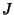## k-Theory

A branch of mathematics which brings together ideas from algebraic geometry, Linear Algebra, and Number Theory. In general, there are two main types of-theory: topological and algebraic.

Topological-theory is the ``true''-theory in the sense that it came first. Topological-theory has to do with Vector Bundles over Topological Spaces. Elements of a-theory are Stable Equivalence classes of Vector Bundles over a Topological Space. You can put a Ring structure on the collection of Stably Equivalent bundles by defining Addition through the Whitney Sum, and Multiplication through the Tensor Product of Vector Bundles. This defines ``the reduced real topological-theory of a space.''

``The reduced-theory of a space'' refers to the same construction, but instead of Real Vector Bundles, Complex Vector Bundles are used. Topological-theory is significant because it forms a generalized Cohomology theory, and it leads to a solution to the vector fields on spheres problem, as well as to an understanding of the-homeomorphism of Homotopy Theory.

Algebraic-theory is somewhat more involved. Swan (1962) noticed that there is a correspondence between the Category of suitably nice Topological Spaces (something like regular Hausdorff Spaces) and C*-Algebra. The idea is to associate to every Space the C*-Algebra of Continuous Maps from that Space to the Reals.

A Vector Bundle over a Space has sections, and these sections can be multiplied by Continuous Functions to the Reals. Under Swan's correspondence, Vector Bundles correspond to modules over the C*-Algebra of Continuous Functions, the Modules being the modules of sections of the Vector Bundle. This study of Modules over C*-Algebra is the starting point of algebraic-theory.

The Quillen-Lichtenbaum Conjecture connects algebraic-theory to Étale cohomology.

Srinivas, V. Algebraic-Theory, 2nd ed. Boston, MA: Birkhäuser, 1995.